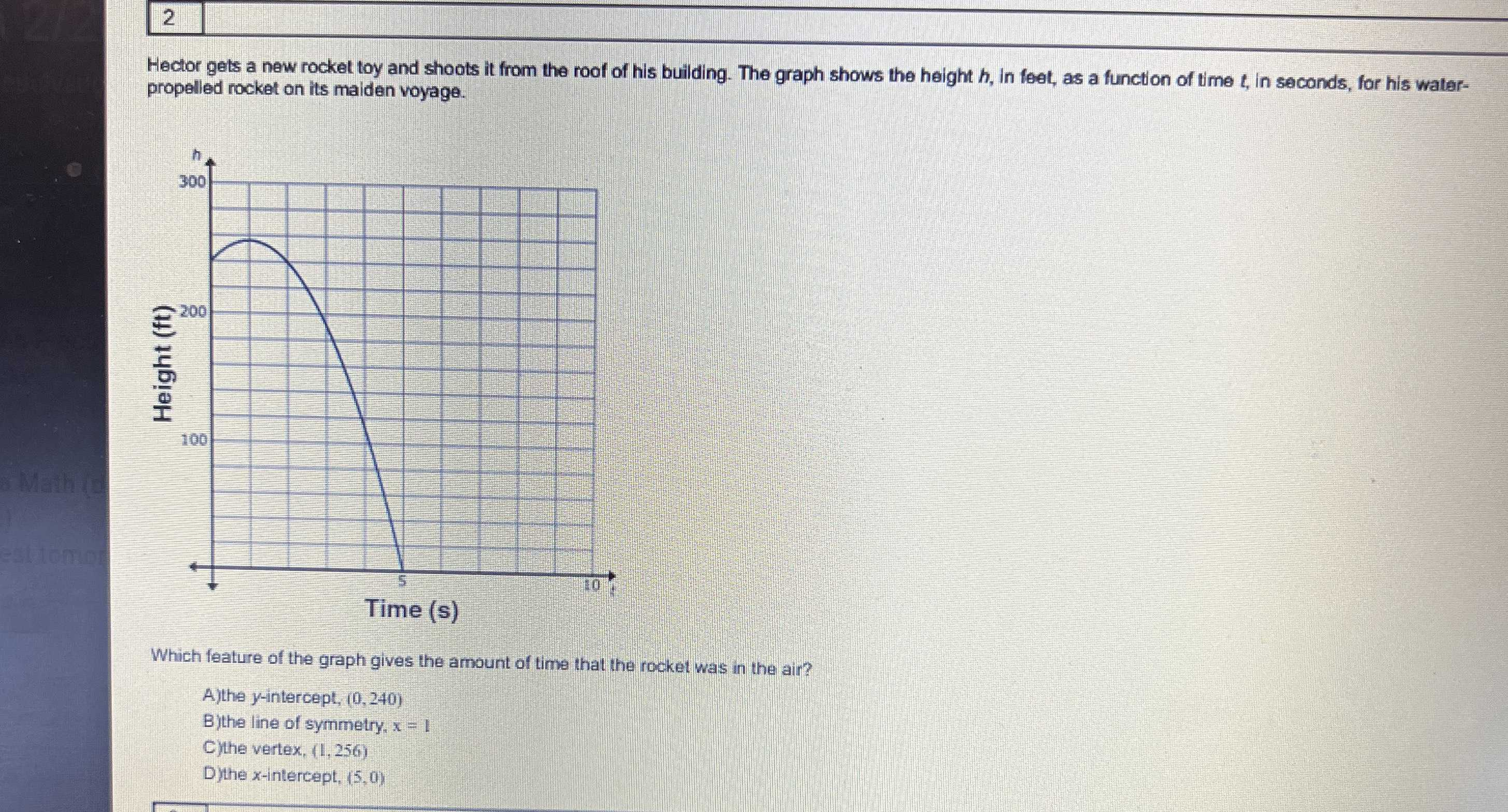### Still have math questions?

Algebra
QuestionWhich feature of the graph gives the amount of time that the rocket was in the air?  A)the $$y$$ -intercept, $$( 0,240 )$$ B)the line of symmetry, $$x = 1$$ C)the vertex, $$( 1,256 )$$ D)the $$x$$ -intercept, $$( 5,0 )$$Hector gets a new rocket toy and shoots it from the roof of his building.

The graph shows the height $$h$$ , in feet, as a function of time $$t$$ , in secends, for his water-  propelled rocket on its maiden voyage.